# Rotations of order 2, with reflections and 10-pointed star

The remaining patterns are numbered 232, which implies that one more further question needs to the answered.

The first group are examples of patterns without a 10-pointed star. An example of two of these are: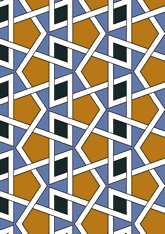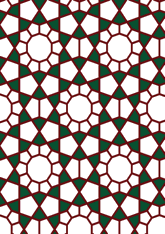The number in this group is 26, which is 11% of the total. Another question is needed - for this see page.
The second group are examples of patterns which have a 10-pointed star having a vertex angle of 72 degree. An example of two of these are: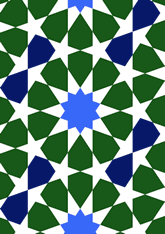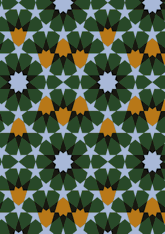The number in this group is 125, which is 53% of the total. Another question is needed - for this see page.
The last group are examples of patterns without the 72 degree 10-pointed star. An example of two of these are: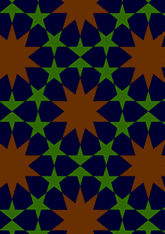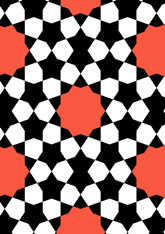The number in this group is 81, which is 34% of the total. Another question is needed - for this see page.

v53

Tiling Search was created by Brian Wichmann, and is maintained by MIT Libraries, through support from the Aga Khan Documentation Center.

Also supported by Performant Software Solutions LLC.